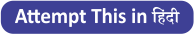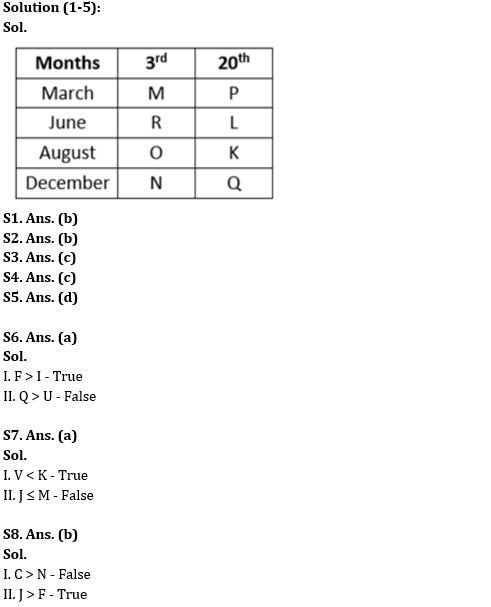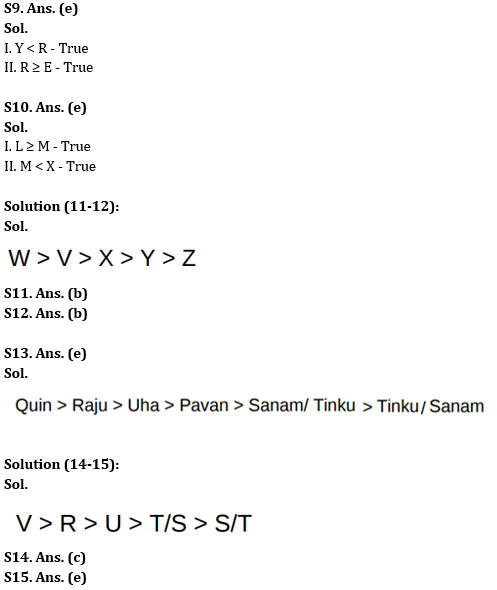Latest Banking jobs   »

# Reasoning Ability Quiz For RBI Grade B/ ECGC PO/ SIDBI Grade A Prelims 2022- 6th May

Directions (1-5): Read the following information carefully and answer the given questions.
Eight people i.e. K, L, M, N, O, P, Q and R have meeting on two different dates i.e. 3rd and 20th of March, June, August, and December of the same year but not necessarily in the same order. L has meeting in the month having less than 31 days. Three people have a meeting between L and Q. The number of people who have a meeting after Q is the same as the number of people who have a meeting before M. One person has a meeting between M and R. Two people have meeting between R and K. P has a meeting before N. Minimum three people have a meeting between P and N.Q1. How many people have meeting between M and L?
(a) Three
(b) Two
(c) None
(d) One
(e) None of these

Q2. Who among the following has meeting on 3rd August?
(a) N
(b) O
(c) K
(d) L
(e) None of these

Q3. How many people have meeting after P?
(a) Five
(b) Seven
(c) Six
(d) Four
(e) None of these

Q4. K has meeting on which among the following date?
(a) 3rd March
(b) 3rd August
(c) 20th August
(d) 20th June
(e) None of these

Q5. If N and R interchange their positions then how many people have meeting between N and Q?
(a) Three
(b) Five
(c) Two
(d) Four
(e) None of these

Directions (6-10): In the following questions assuming the given statement to be true, find which of the conclusion(s) among given conclusions is/are definitely true and then give your answers accordingly.

Q6. Statement: F ≥ G ≥ Y; Q > A > S; Y = U > I; S = D ≤ F
Conclusion:
I. F > I
II. Q > U
(a) Only I is true
(b) Only II is true
(c) Either I or II is true
(d) Neither I nor II is true
(e) Both I and II are true

Q7. Statement: H ≥ G ≥ V; M ≤ L < K; N = B > H; K = J ≥ N
Conclusion:
I. V < K
II. J ≤ M
(a) Only I is true
(b) Only II is true
(c) Either I or II is true
(d) Neither I nor II is true
(e) Both I and II are true

Q8. Statement: H = J ≥ M; D ≤ C = V; G < B = N ≤ H; V > F = G
Conclusion:
I. C > N
II. J > F
(a) Only I is true
(b) Only II is true
(c) Either I or II is true
(d) Neither I nor II is true
(e) Both I and II are true

Q9. Statement: G > Y; E ≤ S = D; F > T = G; D ≤ R = F
Conclusion:
I. Y < R
II. R ≥ E
(a) Only I is true
(b) Only II is true
(c) Either I or II is true
(d) Neither I nor II is true
(e) Both I and II are true

Q10. Statements: N ≥ M < V, P ≤ L ≥ K = N, V ≤ C = X ≥ S
Conclusions:
I. L ≥ M
II. M < X
(a) Only I is true
(b) Only II is true
(c) Either I or II is true
(d) Neither I nor II is true
(e) Both I and II are true

Directions (11-12): Read the following information carefully to answer the questions based on it.
Five persons got different marks. V got less marks than W. X got less marks than V but more marks than Y. Z got the lowest marks among all.

Q11. Who got the highest marks among all?
(a) V
(b) W
(c) X
(d) Y
(e) Cannot be determined

Q12. According to marks, who is exactly in the middle?
(a) Y
(b) X
(c) W
(d) V
(e) Cannot be determined

Q13. Pavan, Quin, Raju, Sanam, Tinku and Uha, have different number of biscuits but not necessarily in the same order. Pavan has more Biscuits than only Sanam and Tinku both but less than that of Quin. Raju has more biscuits than Uha but less than Quin. Who among them have least number of biscuits?
(a) Raju
(b) Sanam
(c) Tinku
(d) Quin
(e) Either Sanam or Tinku

Directions (14-15): Read the following information carefully to answer the questions based on it.
Five friends- R, S, T, U, and V are going to play cricket and have different heights but not necessarily in the same order. U is shorter than R but taller than S. V is taller than R. U is taller than T.

Q14. Who among them is the tallest?
(a) T
(b) R
(c) V
(d) S
(e) None of these

Q15. Who among them is the shortest?
(a) R
(b) U
(c) S
(d) T
(e) Can’t be determined

Solutions#### Congratulations!Union Budget 2023-24: Free PDF